# Sanity check of the new IST and SSD geometries

Figure 1 -- LEFT:  Number of GEANT steps taken vs radius, for y2014a in SL14g.  RIGHT:  Number of GEANT steps taken vs radius for y2014a in DEV (11/13/14).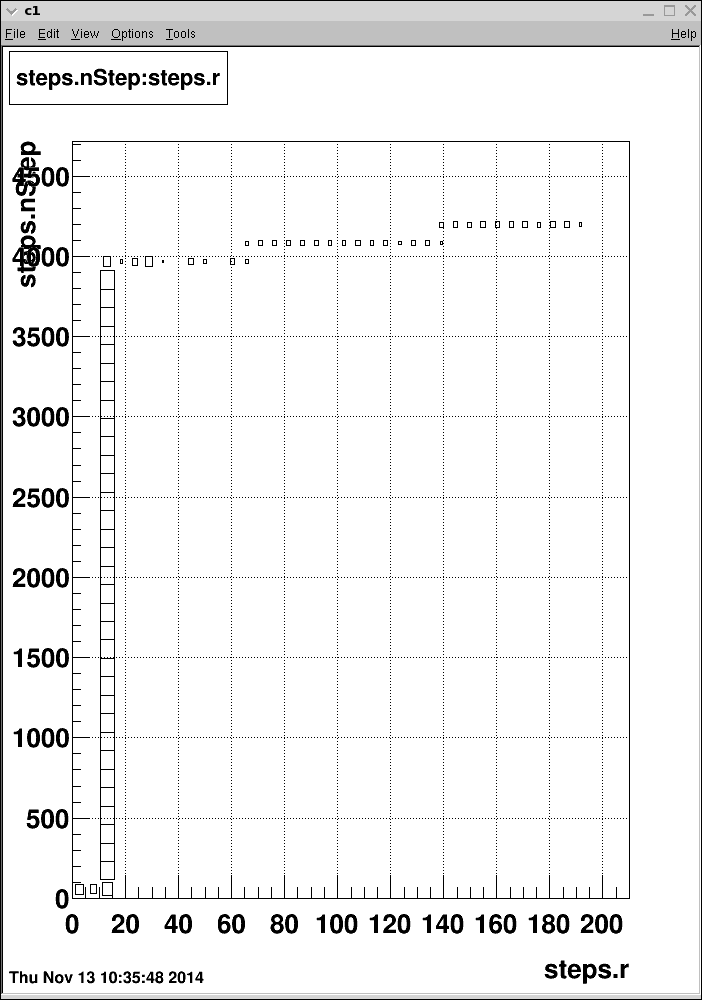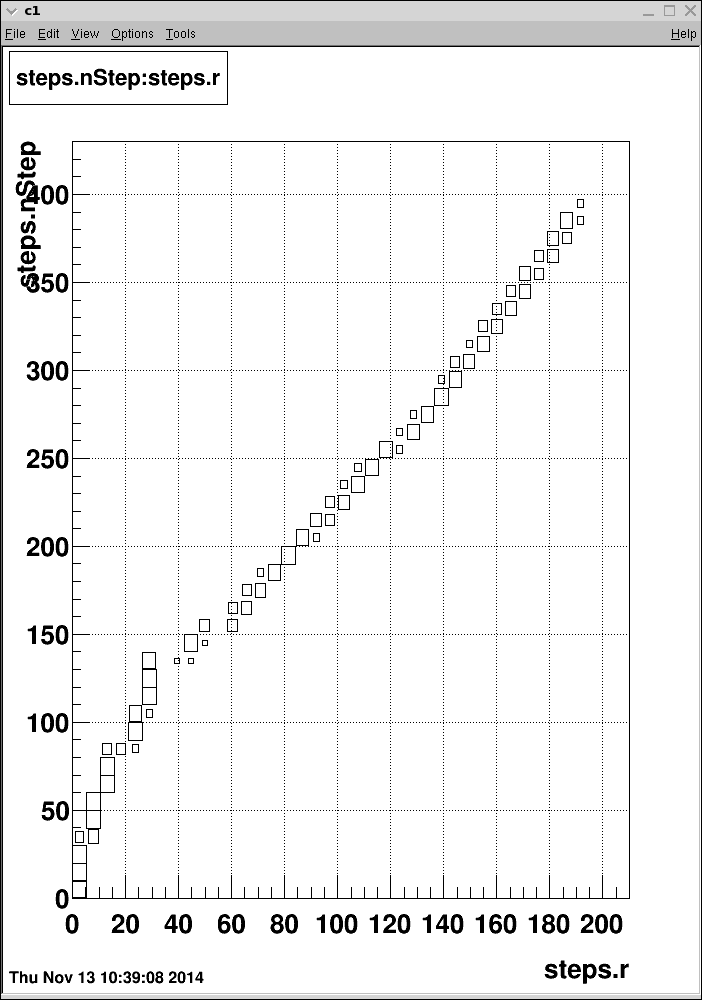Figure 2 -- Accumulated energy loss vs radius.  Comparison between DEV (11/13) and PRO (SL14g).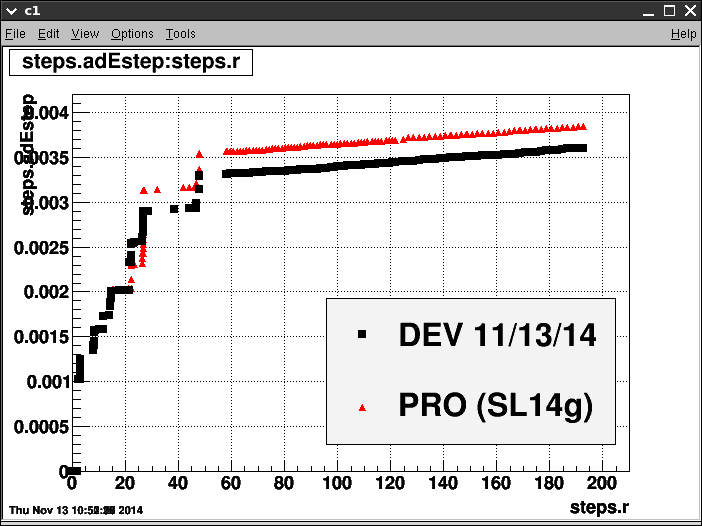Figure 3 -- SFLM Material Distribution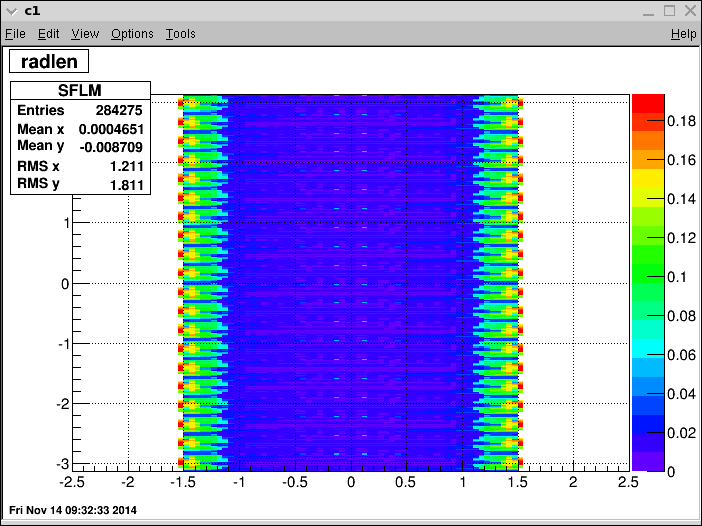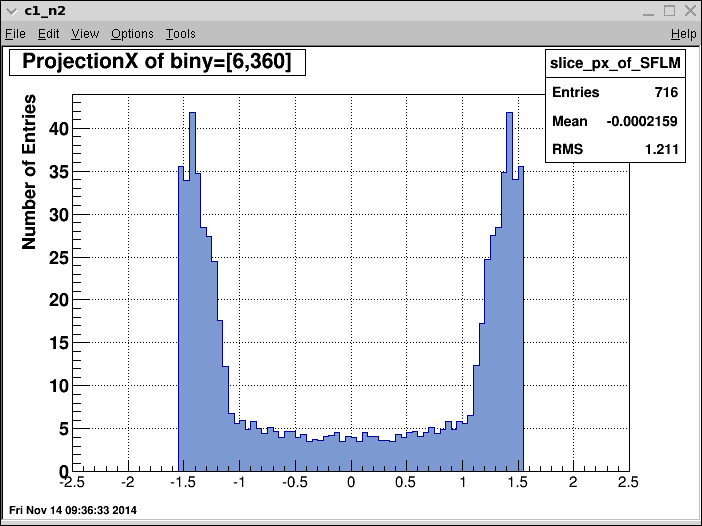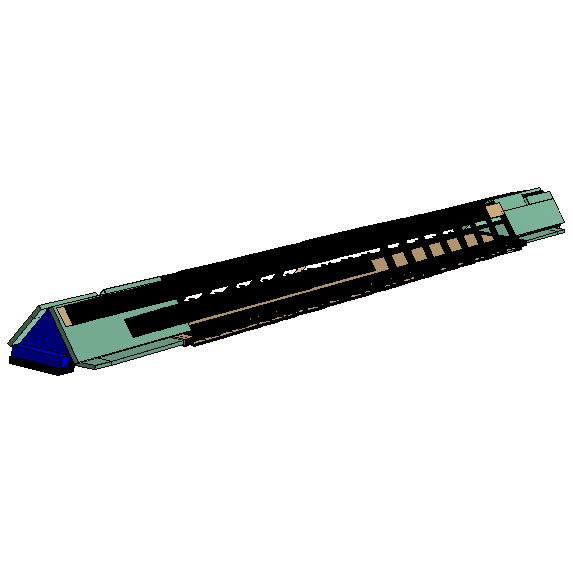Figure 4 -- Obtain density distribution by sampling random points.  Density (arbitray units) vs Z, R and Phi.  Units are arbitrary because I need to divide by the total number of points sampled.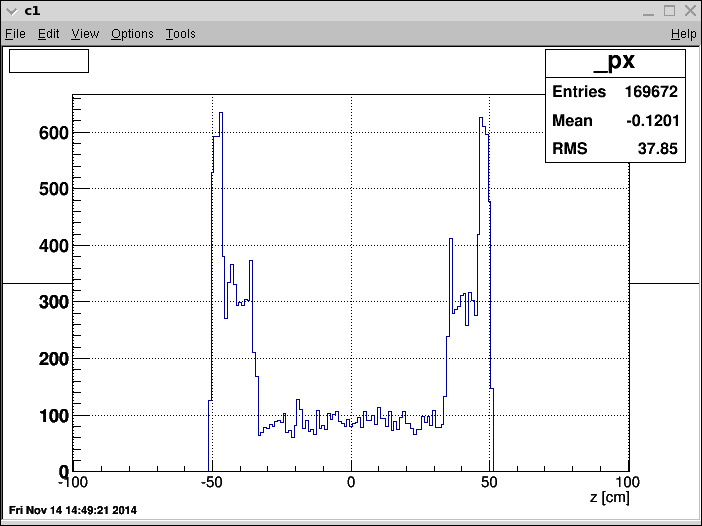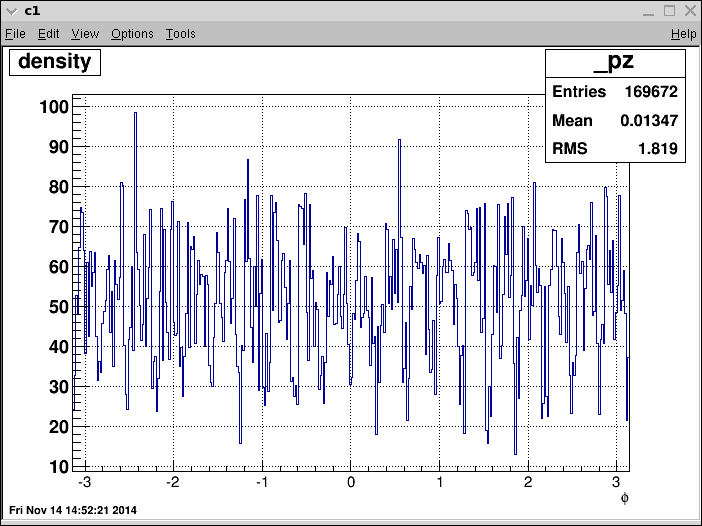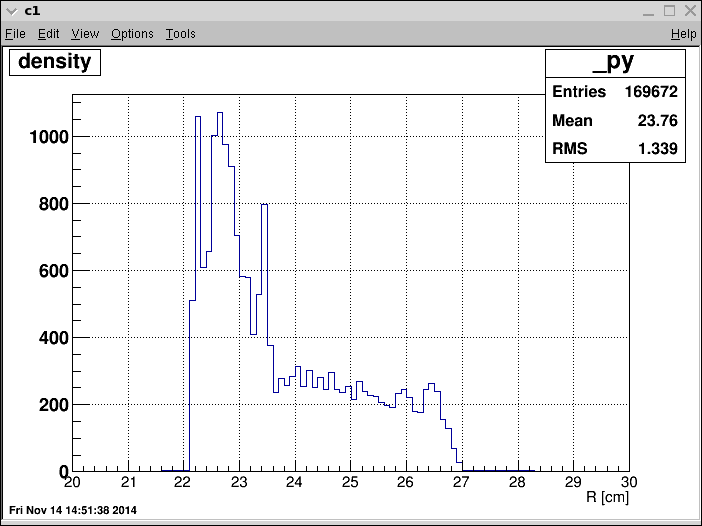Zooming in on the density vs z, I see three regions of interest:

1) -34.0 to +34.0 cm
2) -50.5 to -34.0 cm
3) +34.0 to +50.5 cm

These are approximate, but looks like it gets most of the dense material